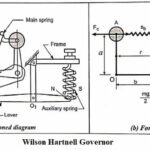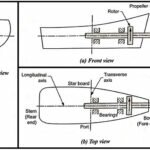# Precessional Angular Motion – theteche.com

• We already know that the angular acceleration is the rate of change of angular velocity. It is a vector quantity and can be represented both in magnitude and direction with the help of right hand screw rule.
• A body rotating in a plane about a fixed axis is subjected to the angular acceleration. But whenever a rotating body changes its axis of rotation, the body will be subjected to both the angular acceleration and another acceleration which is called as gyroscopic acceleration. Therefore the total acceleration of the rotating body is the sum of angular acceleration of the rotating body and gyroscopic acceleration.

#### Derivation

Consider a disc rotating (spinning) in anticlockwise direction, when seen from the front, about an axis OX (known as axis of spin) at an angular speed of ω, as shown in Fig (a). Let the axis of spin move to a new position OX’ in short interval of time δt such that the angular displacement of the axis of spin is δθ and the angular speed of the disc is increased to (ω+δθ).

Using the right hand screw rule, the initial angular velocity of the disc (ω) is represented by vector o𝑥 and the final angular velocity of the disc (ω+δω) is represented by vector o𝑥, as shown in Fig. The vector 𝑥𝑥’ represents the change of angular velocity in time δt i.e., the angular acceleration of the disc.

Resolving vector 𝑥𝑥’ into two mutually perpendicular components, we get

1. Component 𝑥a in the direction of o𝑥, and
2. Component a𝑥 in the direction perpendicular to o𝑥.
##### Component of angular acceleration in the direction of o𝑥 (αt)
Component of angular acceleration along o𝑥 is given by
\begin{aligned} \alpha_{t} &=\frac{x a}{\delta t}=\frac{o a-o x}{\delta t}=\frac{o x^{\prime} \cos \delta \theta-o x}{\delta t} \\ &=\frac{(\omega+\delta \omega) \cos \delta \theta-\omega}{\delta t}=\frac{\omega \cos \delta \theta+\delta \omega \cos \delta \theta-\omega}{\delta t} \end{aligned}
Since δθ is very small, therefore cos δθ = 1, hence we get
$\alpha_{t}=\frac{\omega+\delta \omega-\omega}{\delta t}=\frac{\delta \omega}{\delta t}$
In the limit, when δt → 0
$\alpha_{t}=\underset{\delta t \rightarrow 0}{\mathrm{Lt}}\left(\frac{\delta \omega}{\delta t}\right)=\frac{d \omega}{d t}$
Thus the expression αt = dω/dt represents the change in magnitude of the angular velocity of disc ‘ω’ with respect to time.
##### Component of angular acceleration in the direction perpendicular to o𝑥 (αc)

Component of angular acceleration perpendicular to o𝑥 is given by

$\alpha_{c}=\frac{a x^{\prime}}{\delta t}=\frac{o x^{\prime} \sin \delta \theta}{\delta t}=\frac{(\omega+\delta \omega) \sin \delta \theta}{\delta t}=\frac{\omega \sin \delta \theta+\delta \omega \sin \delta \theta}{\delta t}$

Since δθ is very small, therefore sin δθ = δθ, hence we get

$\alpha_{c}=\frac{\omega \delta \theta+\delta \omega \delta \theta}{\delta t}=\frac{\omega \delta \theta}{\delta t} \quad \ldots[\text { since } \delta \omega \text { and } \delta t \text { are very small, } \therefore \delta \omega \cdot \delta t \approx 0]$

In the limit, when δt →0,

$\alpha_{c}={L t}{\delta t \rightarrow 0} \frac{\omega \delta \theta}{\delta t}=\omega \times \frac{d \theta}{d t}$

αc = ω . ωt

∵ dθ/dt = ωp

Thus the expression αc = ω . ωp represents the change in direction of the axis of spin with respect to time.

##### Total angular acceleration of the disc (α)

Total angular acceleration of the disc is given by

α = vector 𝑥𝑥 = αt + αc

∴ α = dω/dt + ω . ωp

where α= ω . ωp is known as gyroscopic acceleration.
ωp = dθ/dt is known as angular velocity of precession.